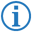## Examples

### Add all the numbers from 1 to 100

`Did you know that`` ``Spike`` ``likes`` ``the number`` ``0``?`
`For every`` ``number`` ``x`` ``from`` ``1`` ``to`` ``100``.``.``.`
`Spike`` ``now likes`` ``Spike`` ``plus`` ``x``.`
`That's what I did``!`

#### Java Equivalent

```double Spike = 0;
for(double x = 1; x < 100; x++){;;
Spike = Spike + x;
}
```

### Iterate through an array

`Did you know that`` ``Berry Punch's favorite thing to say`` ``is`` ``the word`` ``“Cheerwine”``?`
`For every`` ``character`` ``c`` ``in`` ``Berry Punch's favorite thing to say`` ``.``.``.`
`I said`` ``c``.`
`That's what I did``!`

#### Java Equivalent

```char[] Berry_Punchs_favorite_thing_to_say = new char[]{'C','h','e','e','r','w','i','n','e'};
for(char c : Berry_Punchs_favorite_thing_to_say){;;
System.out.println(c);
}
```